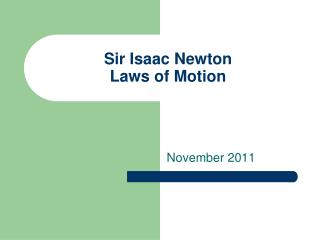DownloadDownload PresentationSir Isaac Newton Laws of Motion

# Sir Isaac Newton Laws of Motion

Télécharger la présentation## Sir Isaac Newton Laws of Motion

- - - - - - - - - - - - - - - - - - - - - - - - - - - E N D - - - - - - - - - - - - - - - - - - - - - - - - - - -
##### Presentation Transcript

1. Sir Isaac NewtonLaws of Motion November 2011

2. 1. Described 3 laws that relate forces to motion 2. Force-a push or a pull, all forces have size and direction Sir Isaac Newton

3. Vocabulary • 1. Friction- a force that opposes motion between 2 objects that are touching • 2. Inertia-tendency of all objects to stay at rest or in motion • 3. Mass- the amount of matter an object is made of

4. Newtown’s 1st Law of Motion “Law of Inertia” • An object at rest remains at rest and an object in motion remains in motion at constant speed and in a straight line unless acted on by an unbalanced force

5. 1. Car suddenly stops and you strain against the seat belt 2. Car turns left and you appear to slide to the right 3. The difficulty of pushing a car that won’t start Examples of Newton’s 1st Law of Motion

6. Examples of Newton’s 1st Law of Motion

7. Examples of Newton’s 1st Law of Motion

8. Write about it! • Write down one example of inertia you see in the room.

9. Bell Work • What is Newton’s 1st Law of Motion? • Is an example of Newton’s 1st Law when a car turns left and you appear to slide to the right? • What is inertia?

10. Newton’s Second Law of Motion • The acceleration of an object depends on the mass of the object and the amount of the force applied

11. Newton’s Second Law of Motion • 1. Force = mass x acceleration F=m x a • 2. Force- a push or pull, all forces have size and direction • 3. Mass- the amount of matter an object is made of • 4. Acceleration-the rate at which velocity changes; and object accelerates if its speed changes, if its direction changes and if both speed and direction changes

12. Examples of Newton’s 2nd Law • 1. Hitting a softball, the harder the hit, the faster the ball goes • 2. Football players and their positions • 3. Loaded versus an unloaded truck

13. Examples of Newton’s 2nd Law

14. Examples of Newton’s 2nd Law

15. Bell Work • What is Newton’s 2nd Law of Motion? • What is an example of Newton’s 2nd Law? • What is friction?

16. Newton’s Third Law of Motion • 1. For every force, there is an equal and opposite force • 2. Action and Reaction

17. Examples of Newton’s 3rd Law • 1. 2 cars hit head on • 2. Jumping out of a boat onto a dock • 3. Astronauts in space